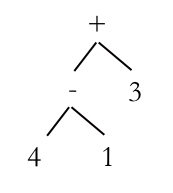# Converting from prefix notation to Abstract Syntax Trees

To convert from prefix notation to an AST, we simply follow this algorithm:

1. The operator becomes a parent
2. The first argument becomes the left child
3. The second argument becomes the right child

If either or both of those arguments are expressions, then we just do the same thing for them. For example, if we have the expression (+ (- 4 1) 3), then the will be the parent, and the left child will be the AST made up from (- 4 1), so  is the parent and 4 and 1 the children. Finally, we have 3 as the right hand child of +.The AST for (+ (- 4 1) 3)

What if we want to go the opposite way and create a prefix expression from an AST? We just start with the root note and apply this algorithm:

1. Write out the operator
2. If the first child is an operator, apply the algorithm to that child, otherwise write it out
3. If the second child is an operator, apply the algorithm to that child, otherwise write it out

Using the AST above, we would first encounter +, followed by – meaning we would apply the algorithm to that subtree, giving us (+ (- 4 1) ..). Next we would apply the final step to 3, which is not an operator, so we write it out.

The exercises below will give you a chance to practice this. Type in your prefix expression and it will calculate the corresponding AST and infix expression.